# New to Qlik Sense

If you’re new to Qlik Sense, start with this Discussion Board and get up-to-speed quickly.

Announcements
QlikWorld 2023, a live, in-person thrill ride. Save \$300 before February 6: REGISTER NOW!
cancel
Showing results for
Did you mean:Not applicable

## Cumulative chart and color coding for calculated measures

Hi,

I am a new user of Qliksense and I have 2 questions:

Question 1:

I am trying to do chart showing cumulative volume and cumulative Gross Margin (see below)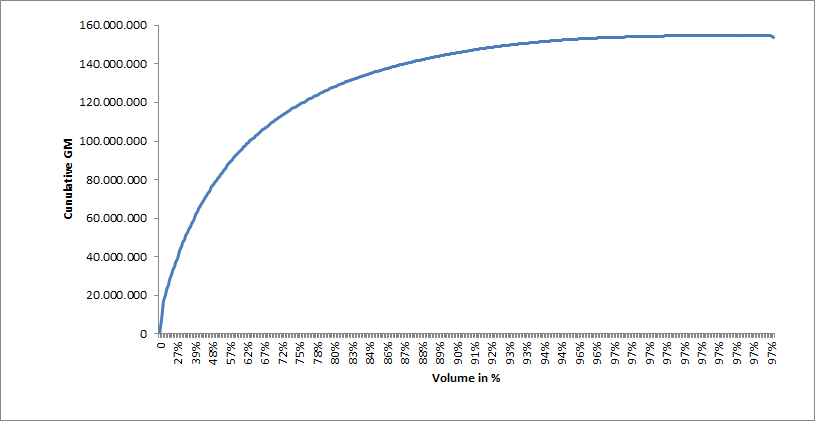Any advise for the expression (formula for cumulative data) and the chart to be used (line chart)???

Question 2:

I have a pivot table with Gross Margin per Liter (Gross Margin divided by Liter). I would like negative value to be in red. I would like to know the formula to add color in Text color expression...

Best regards,

Rodolphe

7 Replies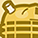Creator

Hi Rodolphe,

Answer #1: Will an adaptation of the article by HIC below work?

Qlik Design Blog : Recipe for a Pareto Analysis | Qlik Community

Answer #2: will the below color expression work?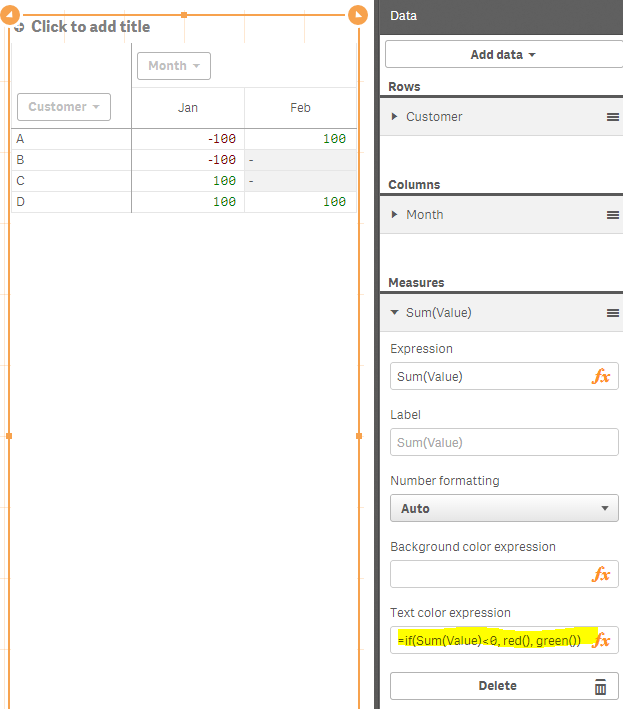Partner - Specialist III

#1: use Rangesum & Above functions with a Line chart

RangeSum(Above(GROSS_MARGIN_EXPRESSION,0,RowNo()))

#2: mhartono solution should work.Not applicable
Author

Thank you

#1: It works for the measure but not for the dimension because a line chart requires a dimension and a measure

When I chose the expression RangeSum(Above(GROSS_MARGIN_EXPRESSION,0,RowNo())) in the dimension field, I got the error message : Invalid Dimension. May be a line chart does not support the Cumulative formula for the Y and X axis.

#2 color coding works for a not calculated measure (Gross Profit), not for a calculated measure (Gross Margin per Liter)

Any suggestion?

Regards,

RodolpheMaster II

Hi Rodolphe,

For the first question, I am assuming that you are looking for something like this:

if((sum([Days In Stock]) / count([Vehicle ID])) < 35,rgb(146,208,80),rgb(255,0,0))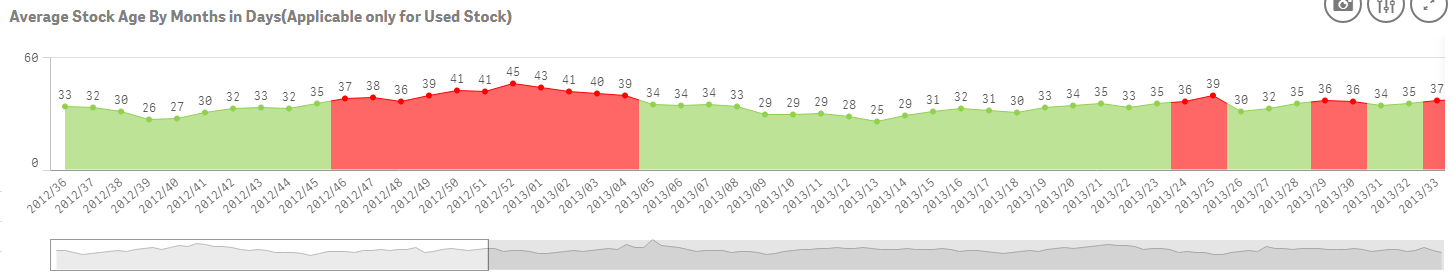And for the second question make use of an expression similar to this:

if((sum([Current Stock Budget])-sum([Stock Value])>0),rgb(146,208,80),rgb(255,0,0))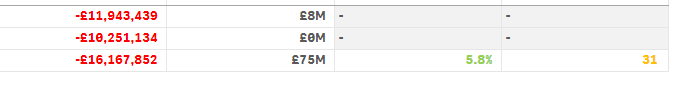Thanks,

Sangram.Not applicable
Author

thanks - #2 is now OK

for the first question I think this is not possible in Qliksense to make a line chart with 2 measures (1 for X axis and 1 for Y axis). Line charts in Qliksense only allow 1 dimension and 1 measure. Will you agree with me?Master II

Hi Rodolphe,

No I am sorry. You can have two lines in a line chart , infact more than 2 check this out: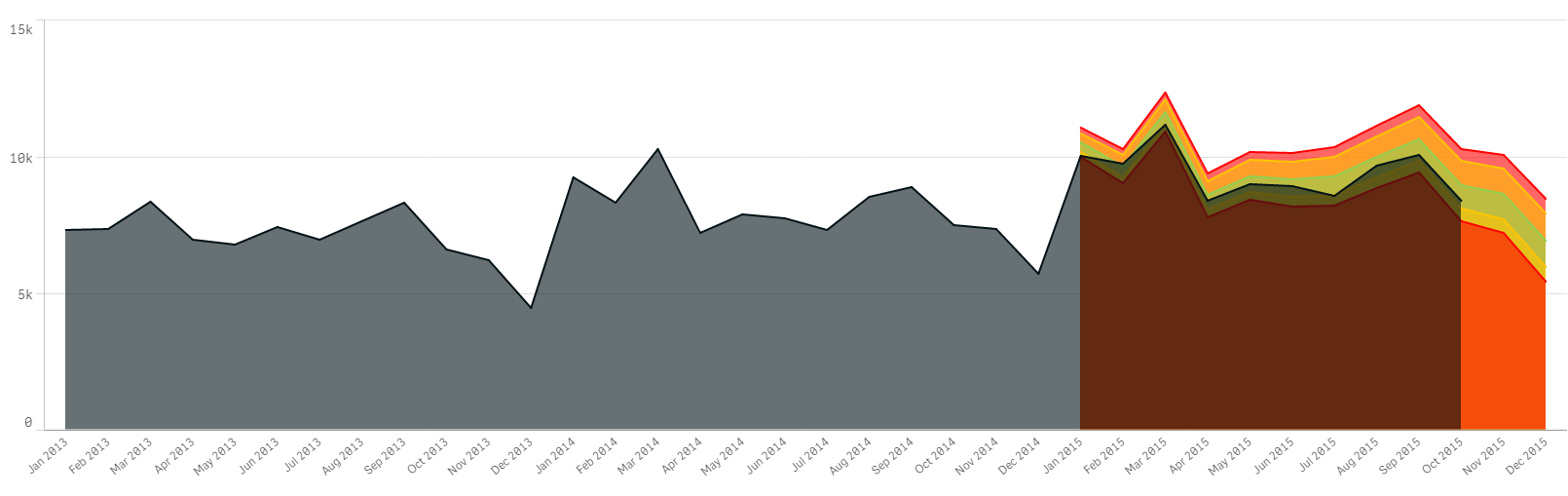Thanks,

Sangram.Master II

Though you have a restriction of 2 dim 1 measure / 1 dim 2 measures, using the pick() function you can implement multi line line chartTags
Community Browser